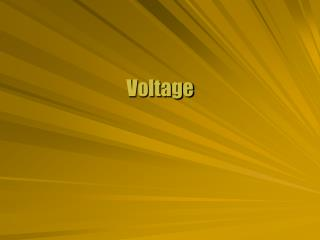DownloadDownload PresentationVoltage

# Voltage

Download Presentation## Voltage

- - - - - - - - - - - - - - - - - - - - - - - - - - - E N D - - - - - - - - - - - - - - - - - - - - - - - - - - -
##### Presentation Transcript

1. Voltage

2. The electric potential is related to the potential energy. Compare to test charge The unit of electric potential is the volt (V). 1 V = 1 J/C q q Volt DV d E F

3. The electric field is most commonly measured in V/m. Show that this is consistent with a measurement in N/C. Use the definitions of N and J to link the two definitions of electric field. 1 N/C = 1 (kg m / s2 ) / C 1 N/C = 1 (kg m) / (s2 C) 1 V/m = 1 (J/C) / m 1 V/m = 1 (N m / C) / m=1 N/C 1 V/m = 1 (kg m2 / s2) / (C m) 1 V/m = 1 (kg m) / (s2 C) Field Measure

4. A cathode ray tube accelerates electrons across a potential of 20 kV. Find the speed of the electrons at the screen. The potential can be converted to an energy. qV = (1.6 x 10-19 C)(2 x 104 V) = 3.2 x 10-15 J. The potential energy becomes kinetic energy. qV = ½ mv2 Solve for the speed v. v = 8.4 x 107 m/s Electric Work

5. A uniform electrical field has the same magnitude and direction at all points. A charge moving parallel to the field lines changes potential by V = Ed. A charge moving perpendicular to the field lines has no change in potential. q q Uniform Field DU d E F

6. There is no field within a conductor. External field neutralized by polarization A test charge at one end moved to the other end would not change potential. All points on a conductor are at the same potential. q q Conductor Potential

7. A voltage source is called a battery. A battery attached to a conducting plate places the same potential across the plates. A uniform electric field exists between the plates. Voltage Source + -

8. A 12-V source is connected to parallel plates 2.0 mm apart. Find the magnitude of the field between the plates. The relationship between the field and voltage is V = Ed. Solve for E = V/d The field is E = (12 V) / (0.002 m) = 6.0 kV/m. Internal Field + - next